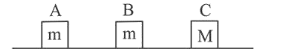# There block A, B and C are lying on a smooth horizontal surface,Question:

There block A, B and C are lying on a smooth horizontal surface, as shown in the figure. A and B have equal masses, $\mathrm{m}$ while $\mathrm{C}$ has mass $\mathrm{M}$. Block $\mathrm{A}$ is given an inital speed $v$ towards B due to which it collides with B perfectly inelastically. The combined mass collides with $\mathrm{C}$,

also perfectly inelastically $\frac{5}{6}$ th of the initial kinetic

energy is lost in whole process. What is value of $\mathrm{M} / \mathrm{m}$ ?1. (1) 5

2. (2) 2

3. (3) 4

4. (4) 3

Correct Option: 3,

Solution:

(3) Kinetic energy of block A

$\mathrm{k}_{1}=\frac{1}{2} \mathrm{mv}_{0}^{2}$

$\therefore$ From principle of linear momentum conservation

$\mathrm{mv}_{0}=(2 \mathrm{~m}+\mathrm{M}) \mathrm{v}_{\mathrm{f}} \Rightarrow \mathrm{v}_{\mathrm{f}}=\frac{\mathrm{mv}_{0}}{2 \mathrm{~m}+\mathrm{M}}$

According to question, of $\frac{5}{6}$ th the initial kinetic energy is lost in whole process.

$\therefore \frac{\mathrm{k}_{\mathrm{i}}}{\mathrm{k}_{\mathrm{f}}}=6 \Rightarrow \frac{\frac{1}{2} \mathrm{mv}_{0}^{2}}{\frac{1}{2}(2 \mathrm{~m}+\mathrm{M})\left(\frac{\mathrm{mv}_{0}}{2 \mathrm{~m}+\mathrm{M}}\right)^{2}}=6$

According to question, of $\frac{5}{6}$ th the initial kinetic energy is lost in whole process.

$\therefore \frac{\mathrm{k}_{\mathrm{i}}}{\mathrm{k}_{\mathrm{f}}}=6 \Rightarrow \frac{\frac{1}{2} \mathrm{mv}_{0}^{2}}{\frac{1}{2}(2 \mathrm{~m}+\mathrm{M})\left(\frac{\mathrm{mv}_{0}}{2 \mathrm{~m}+\mathrm{M}}\right)^{2}}=6$

$\Rightarrow \frac{2 m+M}{m}=6 \therefore \frac{M}{m}=4$